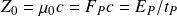# Vacuum impedance

Vacuum impedance relates the strengths of electric and magnetic potentials acting on radiation in the vacuum. The translation of electromagnetic units into MKS units gives magnitude and dimensions to the vacuum impedance of Planck energy to Planck time.

Vacuum impedance is derived from the product of the magnetic constant and the speed of light. In MKS units, the formula isThe elementary form of vacuum impedance is

## Electromagnetic unit conversion

Calculations using the vacuum impedance in MKS units require a unit conversion. The following conversion factor translates units of ohm into meters, kilograms, and seconds.

Full table of electromagnetic unit conversions

Vacuum impedance